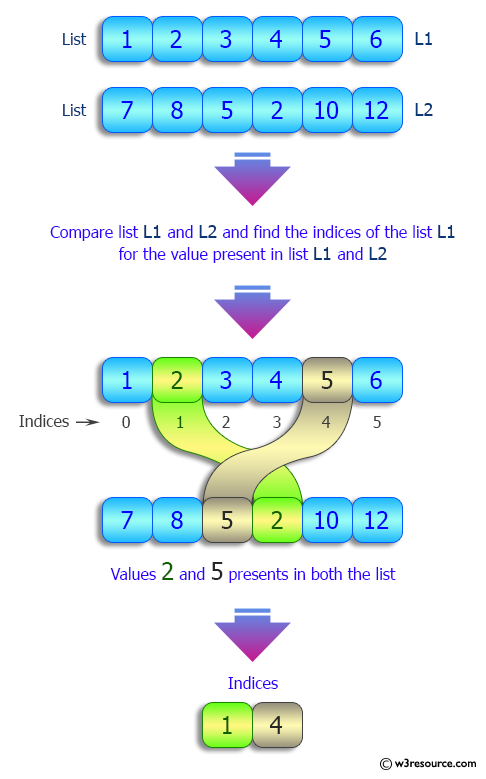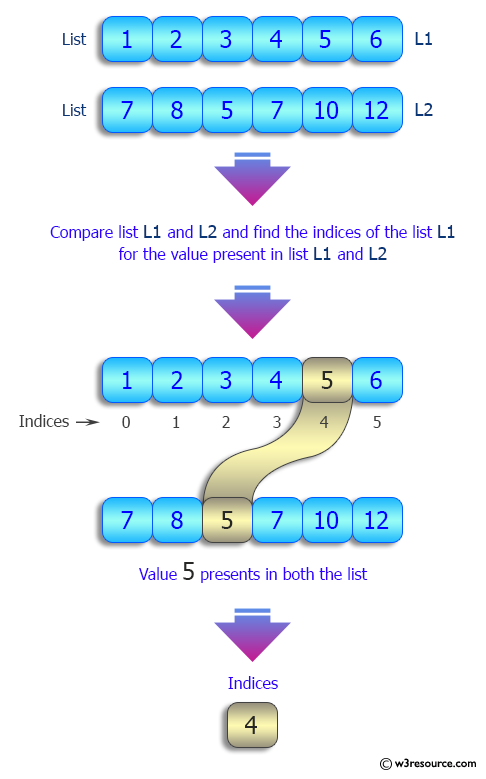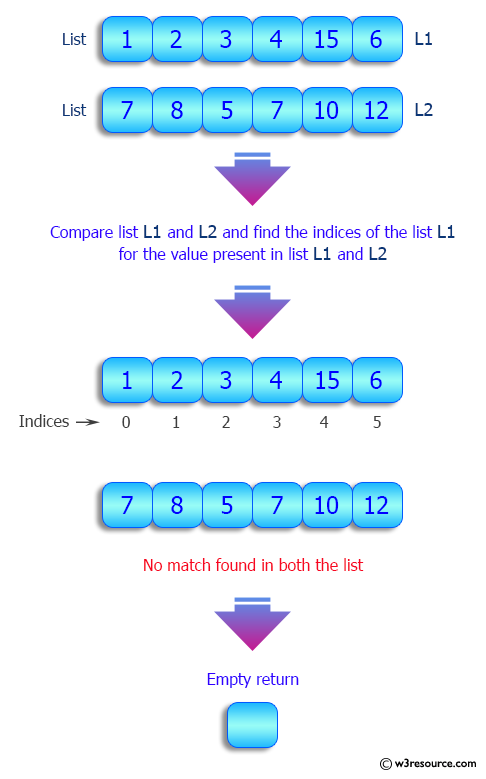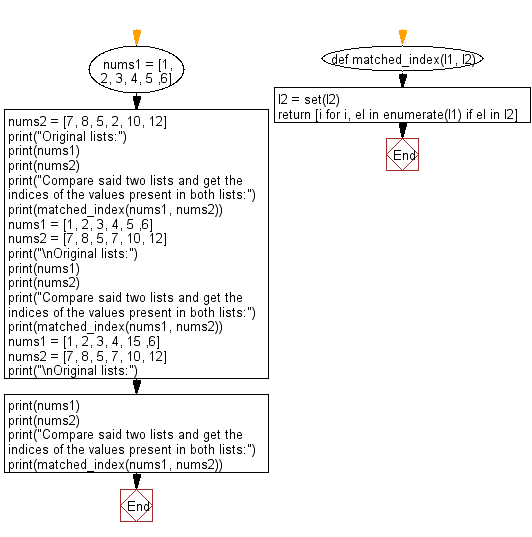﻿ Python: Compare two given lists and find the indices of the values present in both lists - w3resource# Python: Compare two given lists and find the indices of the values present in both lists

## Python List: Exercise - 198 with Solution

Write a Python program to compare two given lists and find the indices of the values present in both lists.

Sample Solution:

Python Code:

``````def matched_index(l1, l2):
l2 = set(l2)
return [i for i, el in enumerate(l1) if el in l2]

nums1 = [1, 2, 3, 4, 5 ,6]
nums2 = [7, 8, 5, 2, 10, 12]
print("Original lists:")
print(nums1)
print(nums2)
print("Compare said two lists and get the indices of the values present in both lists:")
print(matched_index(nums1, nums2))

nums1 = [1, 2, 3, 4, 5 ,6]
nums2 = [7, 8, 5, 7, 10, 12]
print("\nOriginal lists:")
print(nums1)
print(nums2)
print("Compare said two lists and get the indices of the values present in both lists:")
print(matched_index(nums1, nums2))

nums1 = [1, 2, 3, 4, 15 ,6]
nums2 = [7, 8, 5, 7, 10, 12]
print("\nOriginal lists:")
print(nums1)
print(nums2)
print("Compare said two lists and get the indices of the values present in both lists:")
print(matched_index(nums1, nums2))
```
```

Sample Output:

```Original lists:
[1, 2, 3, 4, 5, 6]
[7, 8, 5, 2, 10, 12]
Compare said two lists and get the indices of the values present in both lists:
[1, 4]

Original lists:
[1, 2, 3, 4, 5, 6]
[7, 8, 5, 7, 10, 12]
Compare said two lists and get the indices of the values present in both lists:


Original lists:
[1, 2, 3, 4, 15, 6]
[7, 8, 5, 7, 10, 12]
Compare said two lists and get the indices of the values present in both lists:
[]
```

Pictorial Presentation:Flowchart:## Visualize Python code execution:

The following tool visualize what the computer is doing step-by-step as it executes the said program:

Python Code Editor:

Have another way to solve this solution? Contribute your code (and comments) through Disqus.

What is the difficulty level of this exercise?

Test your Python skills with w3resource's quiz

﻿

## Python: Tips of the Day

Floor Division:

When we speak of division we normally mean (/) float division operator, this will give a precise result in float format with decimals.

For a rounded integer result there is (//) floor division operator in Python. Floor division will only give integer results that are round numbers.

```print(1000 // 300)
print(1000 / 300)```

Output:

```3
3.3333333333333335```